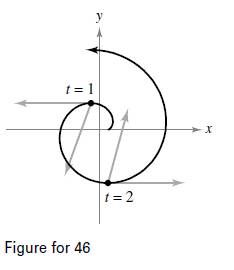Chapter 12.4, Problem 50E

Chapter
Section
Textbook Problem
1 views

# Motion Along an Involute of a Circle The figure shows a particle moving along a path modeled by r ( t ) = 〈 cos π t + π t sin π t , sin π t − π t cos π t 〉 .The figure also shows the vectors v(t) and a(t) for t = 1 and t = 2 .(a) Find a T and a N at t = 1 and t = 2 .(b) Determine whether the speed of the particle is increasing or decreasing at each of the indicated values of t. Give reasons for your answers.(a)

To determine

To Calculate: The value of the tangential and normal components of acceleration as, aT and aN at t=1 and t=2 if the position vector is,

r(t)= cosπt+πtsinπt, sinπtπtcosπt.

Explanation

Given:

The provided position vector is, r(t)= cosπt+πtsinπt, sinπtπtcosπt.

Formula Used:

The tangential velocity and tangential acceleration are:

v(t)=dr(t)dt and a(t)=dv(t)dt.

Calculation:

The velocity is calculated as shown below,

v(t)=r(t)=πsinπt+πsinπt+π2tcosπt, πcosπtπcosπt+π2sinπt= π2tcosπt,π2tsinπt

Now, find the magnitude of the velocity vector to find speed of the particle as shown below,

v(t)= π2t …… (1)

Now, find the acceleration vector of the particle as calculated below,

a(t)= r(t)=π2cosπtπ3tsinπt, π2sinπt+π3t cosπt

Now, find the tangential component of acceleration as shown below,

aT=v·av=π2tcosπt,π2tsinπt·π2cosπtπ3tsinπt, π2sinπt+π3t cosπtπ2t=π4tcos2πtπ5tcosπtsinπt+π4tsin2πt+π5t2sinπtcosπtπ2t=π4t(cos2πt+sin2πt)π2t

Since, cos2π+sin2πt=1

The value is found as shown below,

aT=π2

At t=1 and t=2 the tangent vector is aT=π2

(b)

To determine
Whether the speed of particle is increasing or decreasing at each of the indicated value of t from t=1 to t=2 if the position vector is,

r(t)= cosπt+πtsinπt, sinπtπtcosπt.

### Still sussing out bartleby?

Check out a sample textbook solution.

See a sample solution

#### The Solution to Your Study Problems

Bartleby provides explanations to thousands of textbook problems written by our experts, many with advanced degrees!

Get Started

#### Convert from degrees to radians. 1. 210

Single Variable Calculus: Early Transcendentals, Volume I

#### Evaluate the expression sin Exercises 116. (23)2

Finite Mathematics and Applied Calculus (MindTap Course List)

#### Use a Table of Integrals to evaluate .

Study Guide for Stewart's Single Variable Calculus: Early Transcendentals, 8th

#### For what values of p does the series converge?

Study Guide for Stewart's Multivariable Calculus, 8th